Add alternating background color along the y-axis. The geom takes default aesthetics odd and even that receive color codes.

geom_stripped_rows(
mapping = NULL,
data = NULL,
stat = "identity",
position = "identity",
...,
show.legend = NA,
inherit.aes = TRUE,
xfrom = -Inf,
xto = Inf,
width = 1,
nudge_y = 0
)

geom_stripped_cols(
mapping = NULL,
data = NULL,
stat = "identity",
position = "identity",
...,
show.legend = NA,
inherit.aes = TRUE,
yfrom = -Inf,
yto = Inf,
width = 1,
nudge_x = 0
)

## Arguments

mapping

Set of aesthetic mappings created by aes(). If specified and inherit.aes = TRUE (the default), it is combined with the default mapping at the top level of the plot. You must supply mapping if there is no plot mapping.

data

The data to be displayed in this layer. There are three options:

If NULL, the default, the data is inherited from the plot data as specified in the call to ggplot().

A data.frame, or other object, will override the plot data. All objects will be fortified to produce a data frame. See fortify() for which variables will be created.

A function will be called with a single argument, the plot data. The return value must be a data.frame, and will be used as the layer data. A function can be created from a formula (e.g. ~ head(.x, 10)).

stat

The statistical transformation to use on the data for this layer, either as a ggproto Geom subclass or as a string naming the stat stripped of the stat_ prefix (e.g. "count" rather than "stat_count")

position

Position adjustment, either as a string naming the adjustment (e.g. "jitter" to use position_jitter), or the result of a call to a position adjustment function. Use the latter if you need to change the settings of the adjustment.

...

Other arguments passed on to layer(). These are often aesthetics, used to set an aesthetic to a fixed value, like colour = "red" or size = 3. They may also be parameters to the paired geom/stat.

show.legend

logical. Should this layer be included in the legends? NA, the default, includes if any aesthetics are mapped. FALSE never includes, and TRUE always includes. It can also be a named logical vector to finely select the aesthetics to display.

inherit.aes

If FALSE, overrides the default aesthetics, rather than combining with them. This is most useful for helper functions that define both data and aesthetics and shouldn't inherit behaviour from the default plot specification, e.g. borders().

xfrom, xto

limitation of the strips along the x-axis

width

width of the strips

yfrom, yto

limitation of the strips along the y-axis

nudge_x, nudge_y

horizontal or vertical adjustment to nudge strips by

## Value

A ggplot2 plot with the added geometry.

## Examples

data(tips, package = "reshape")

library(ggplot2)
p <- ggplot(tips) +
aes(x = time, y = day) +
geom_count() +
theme_light()

p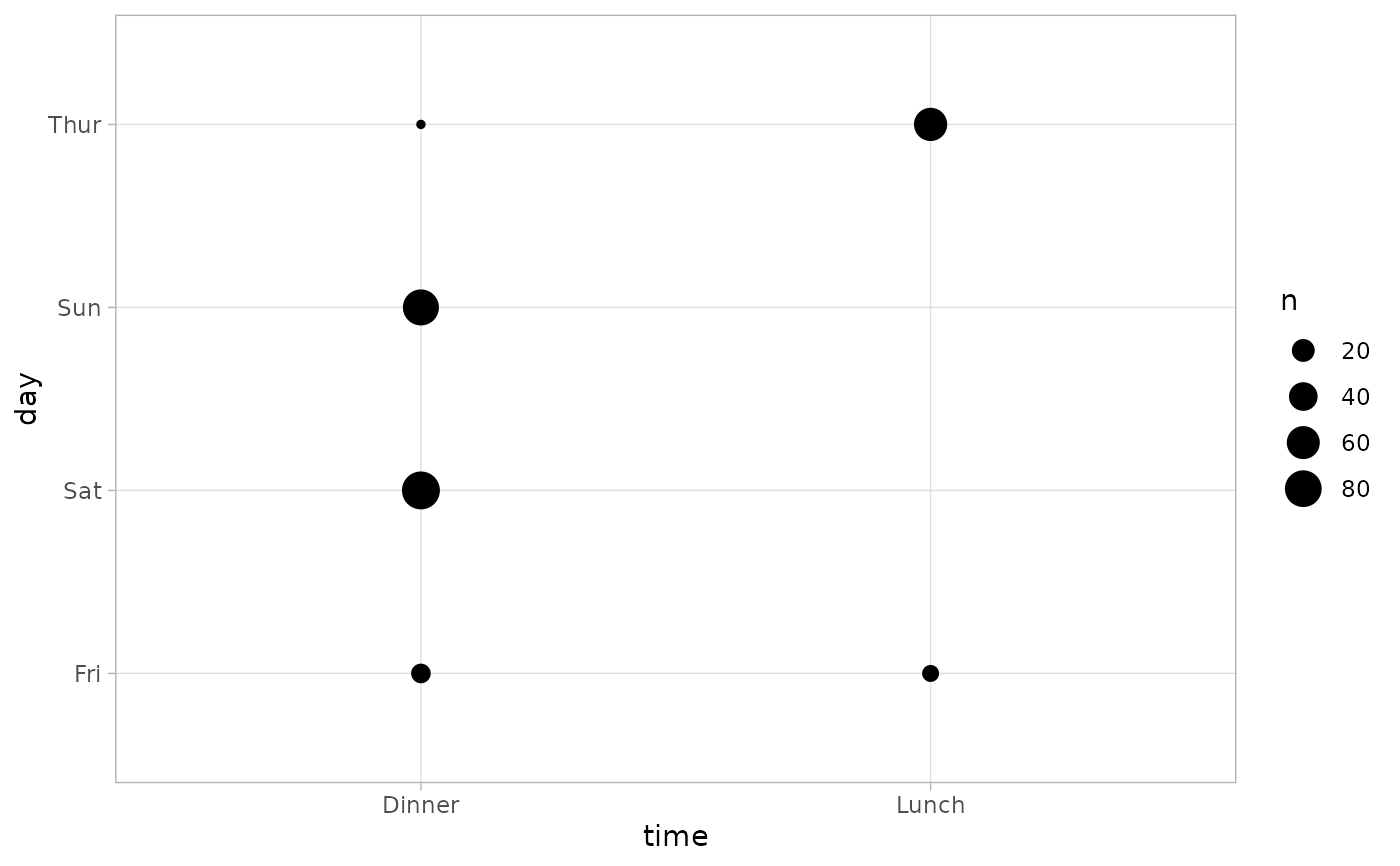p + geom_stripped_rows()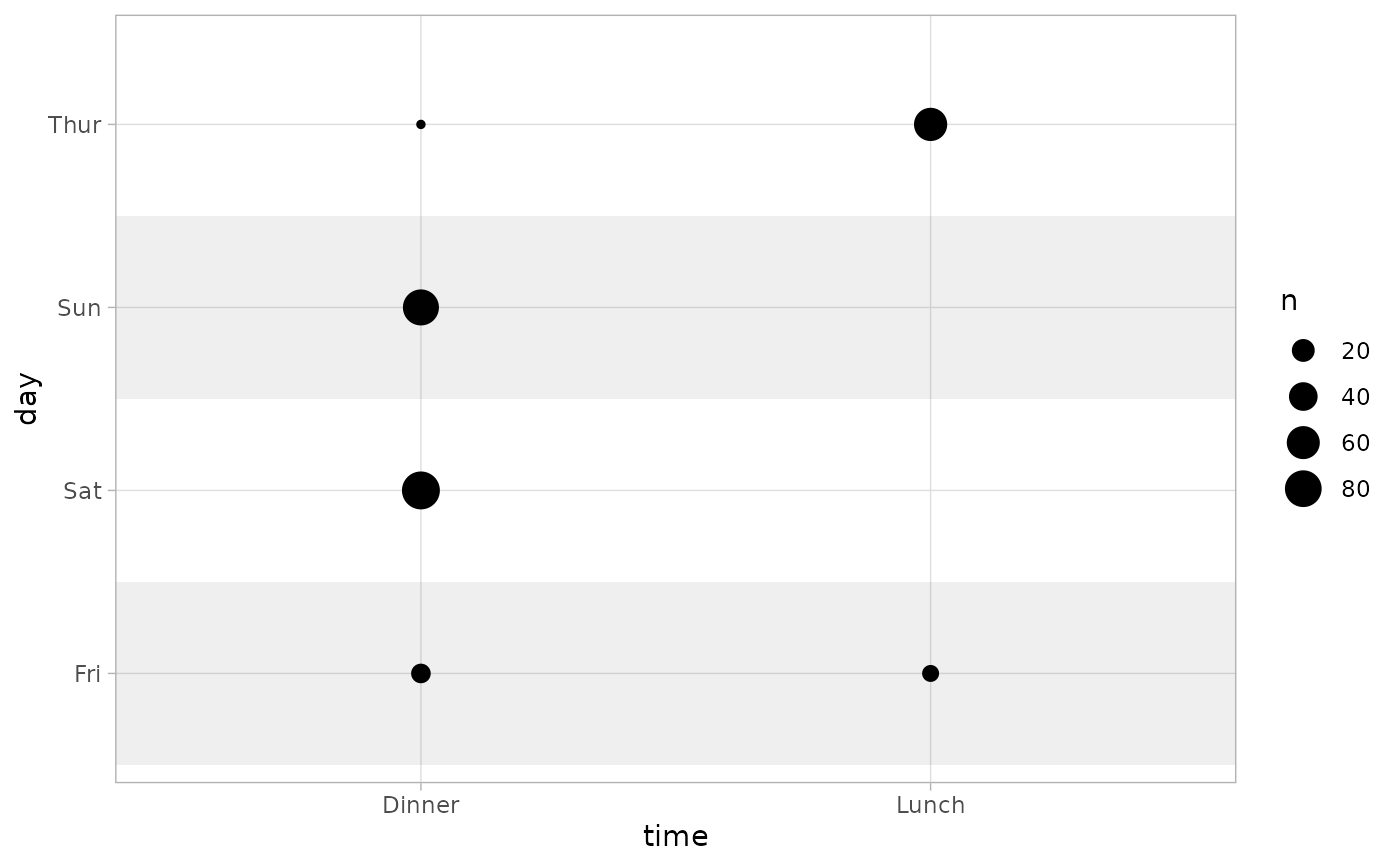p + geom_stripped_cols()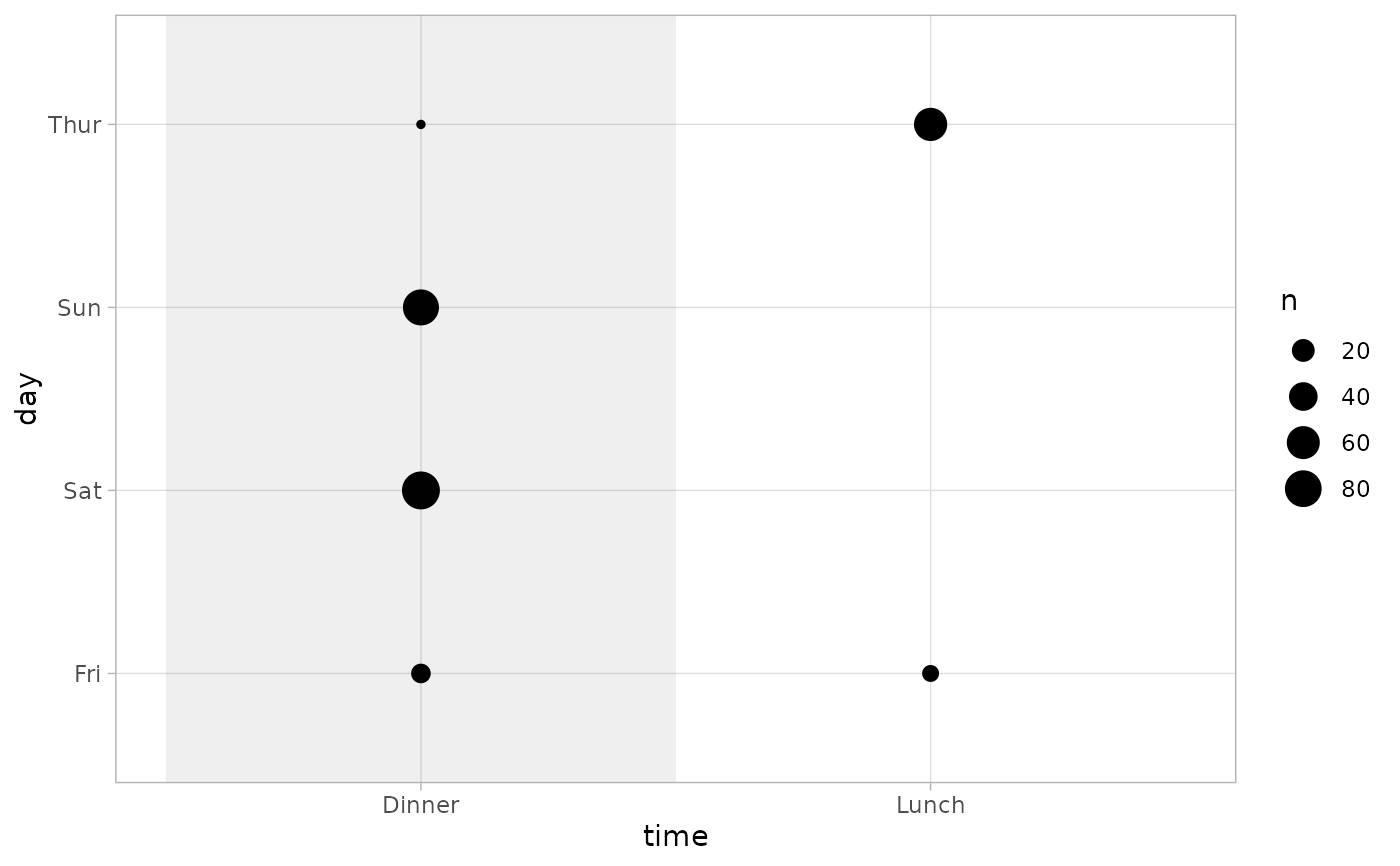p + geom_stripped_rows() + geom_stripped_cols()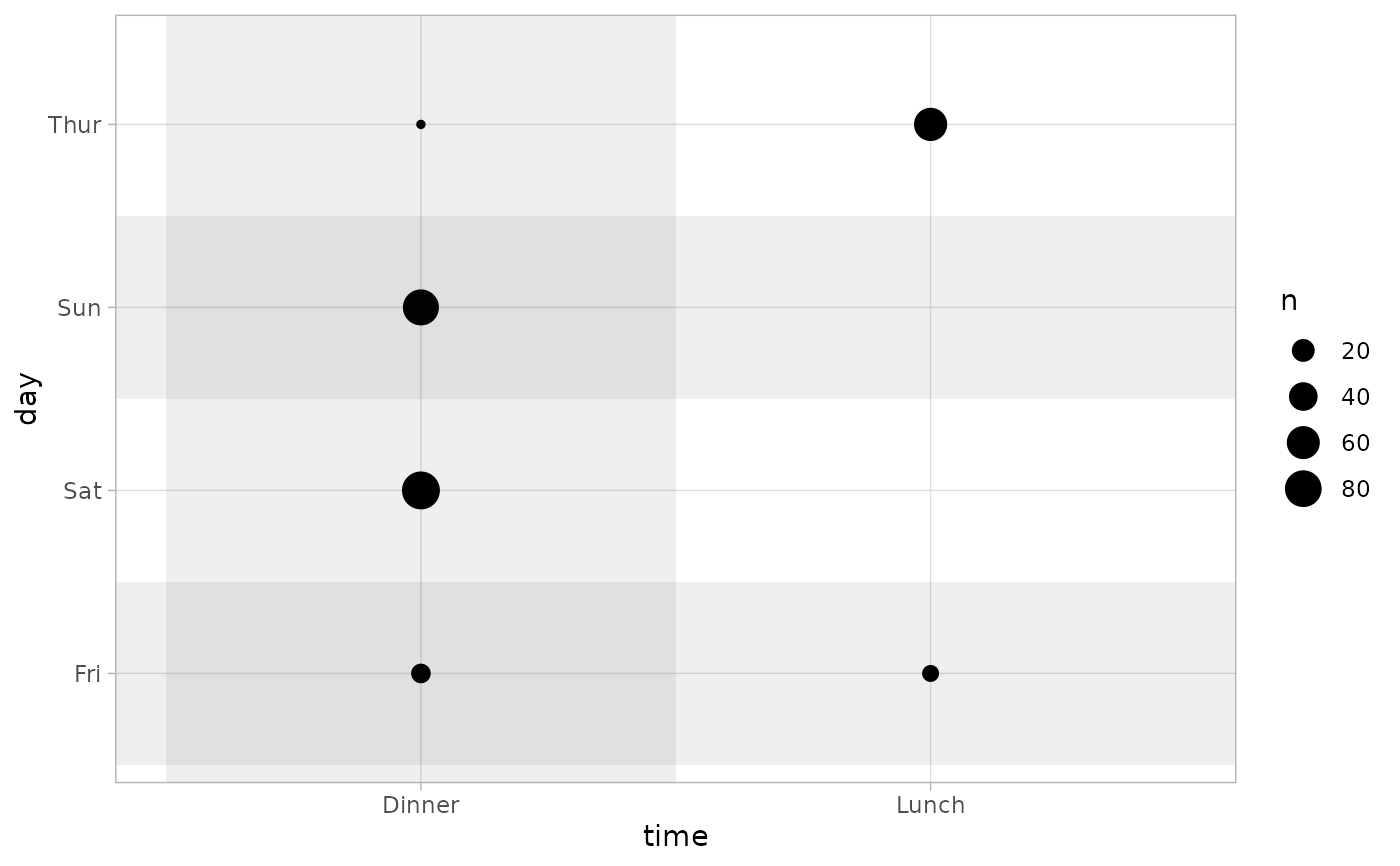# \donttest{
p <- ggplot(tips) +
aes(x = total_bill, y = day) +
geom_count() +
theme_light()
p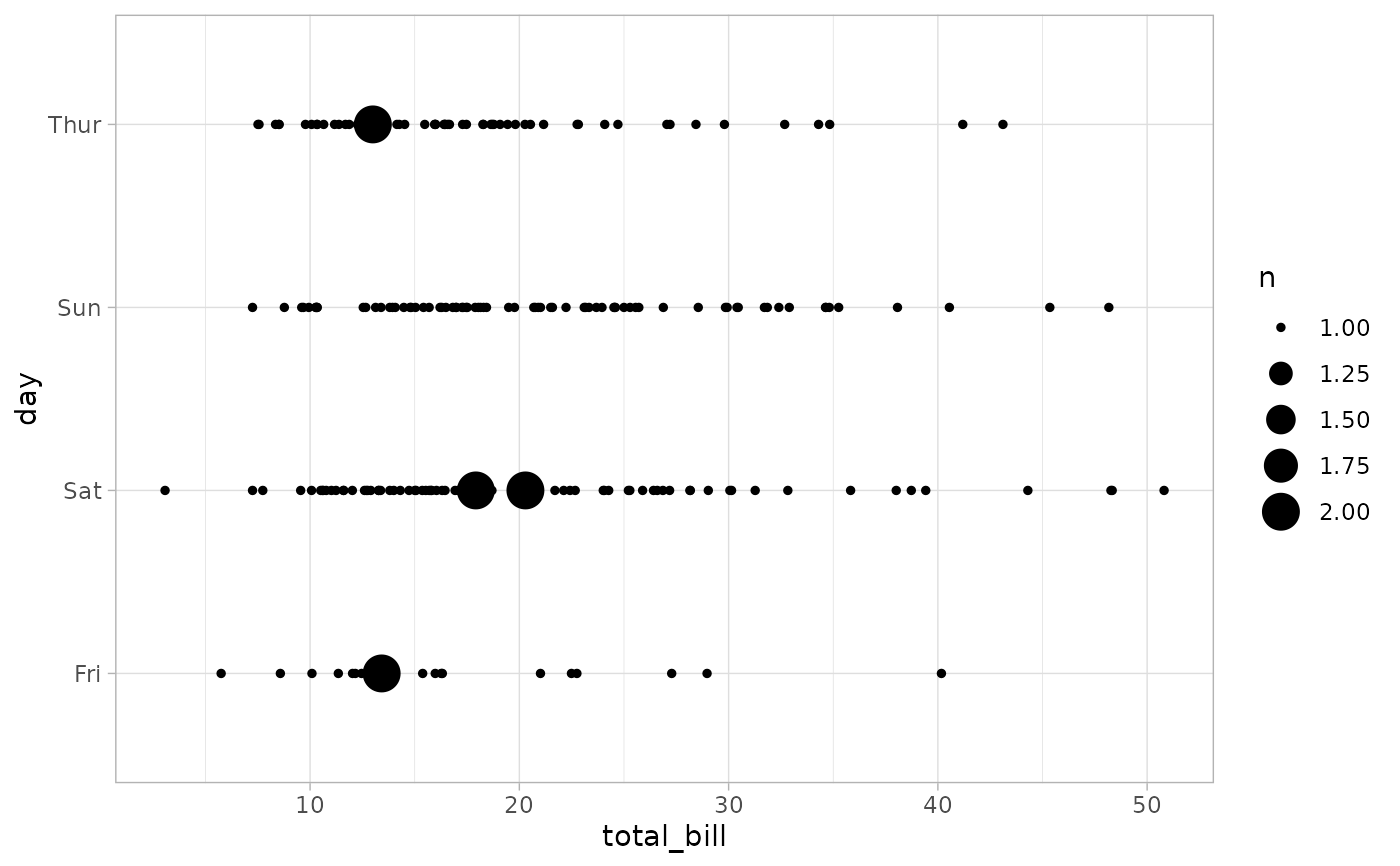p + geom_stripped_rows()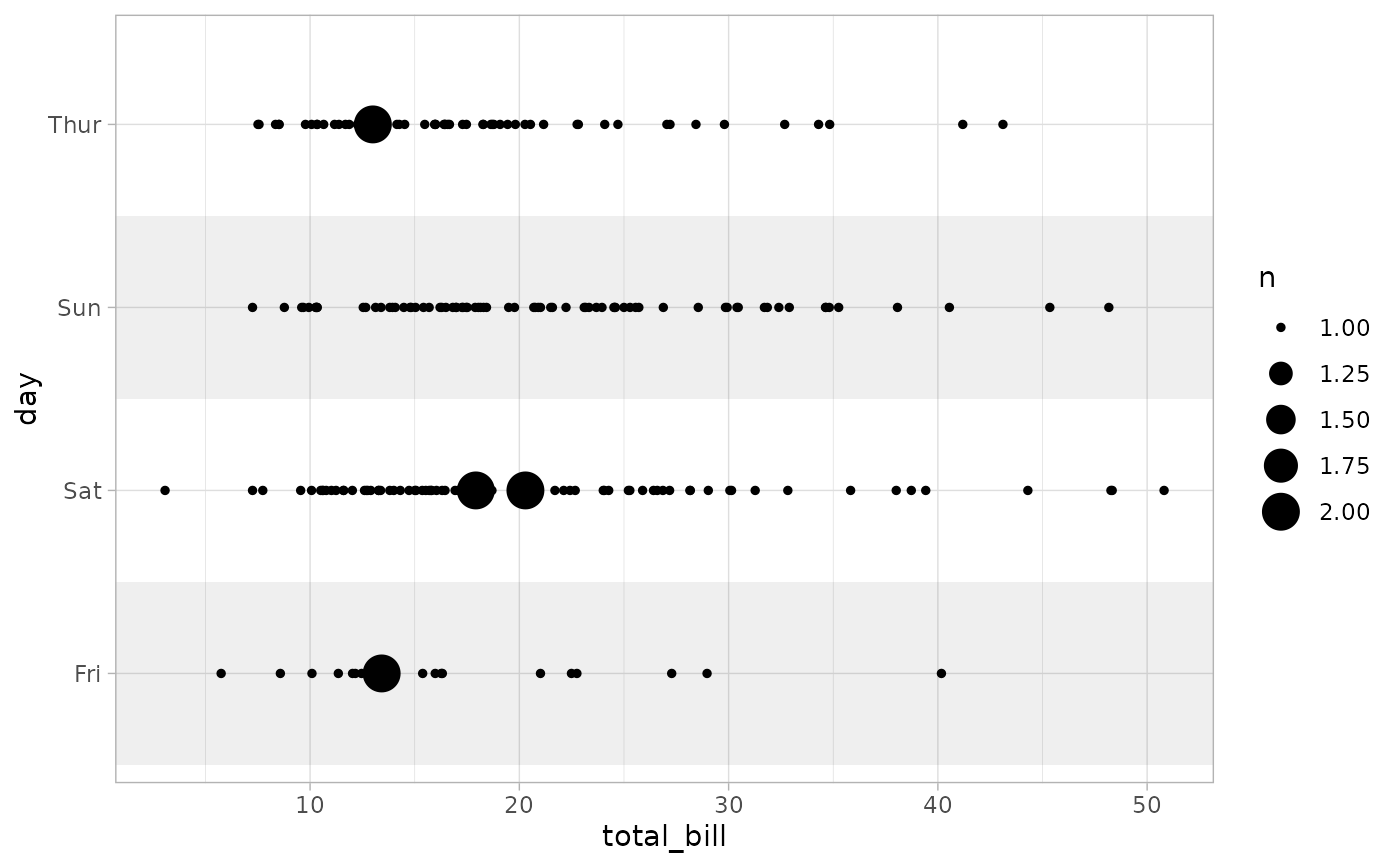p + geom_stripped_rows() + scale_y_discrete(expand = expansion(0, 0.5))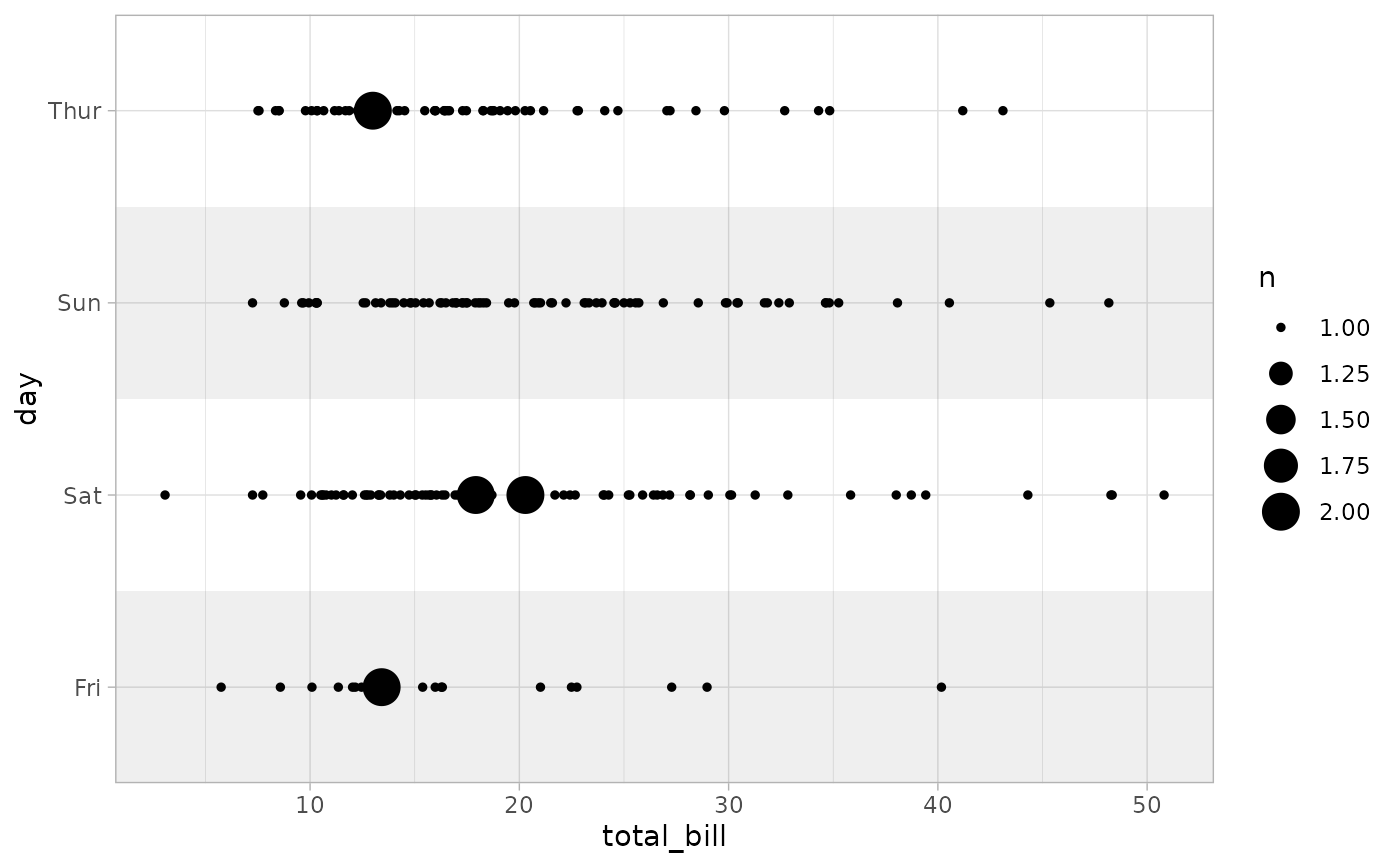p + geom_stripped_rows(xfrom = 10, xto = 35)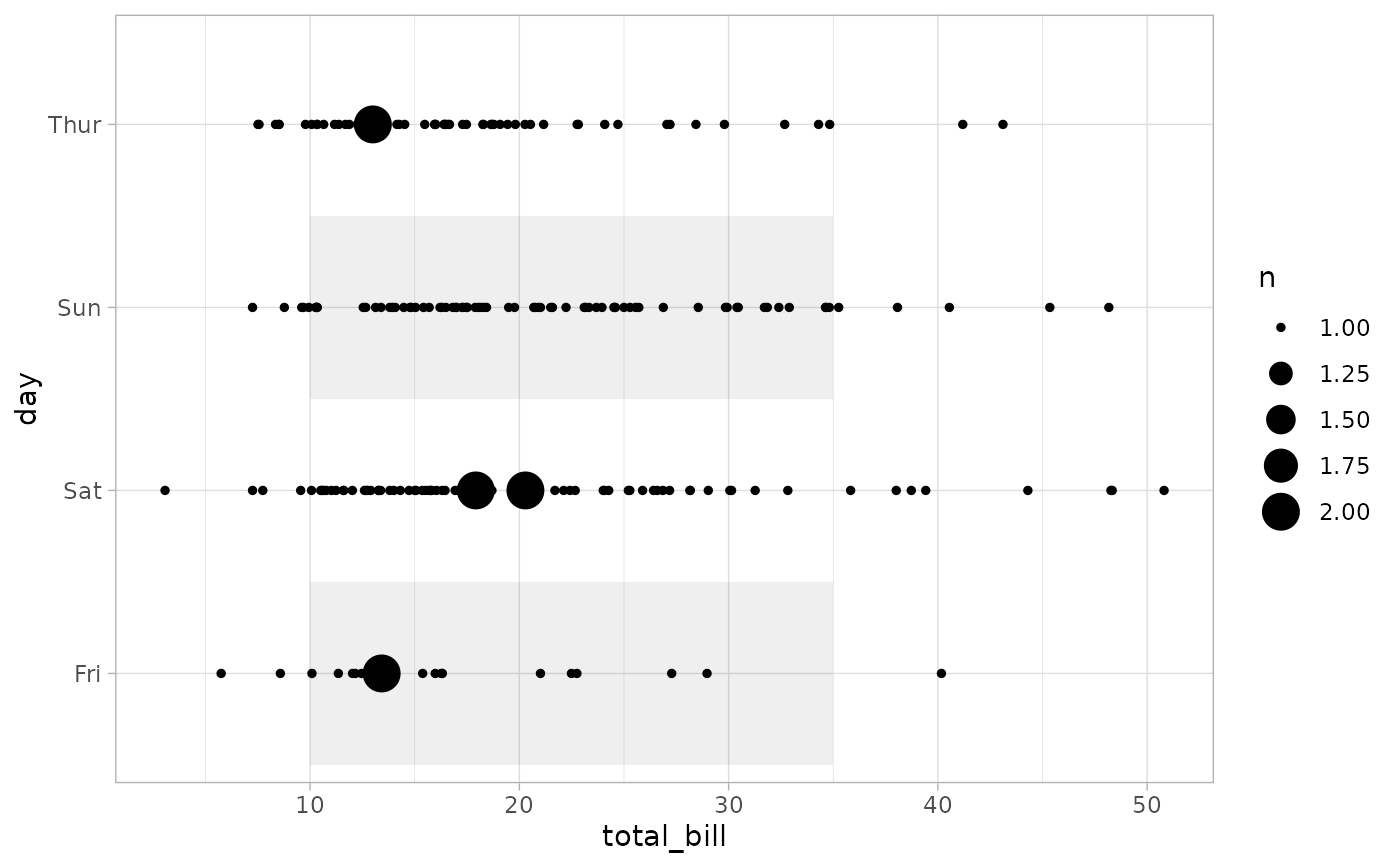p + geom_stripped_rows(odd = "blue", even = "yellow")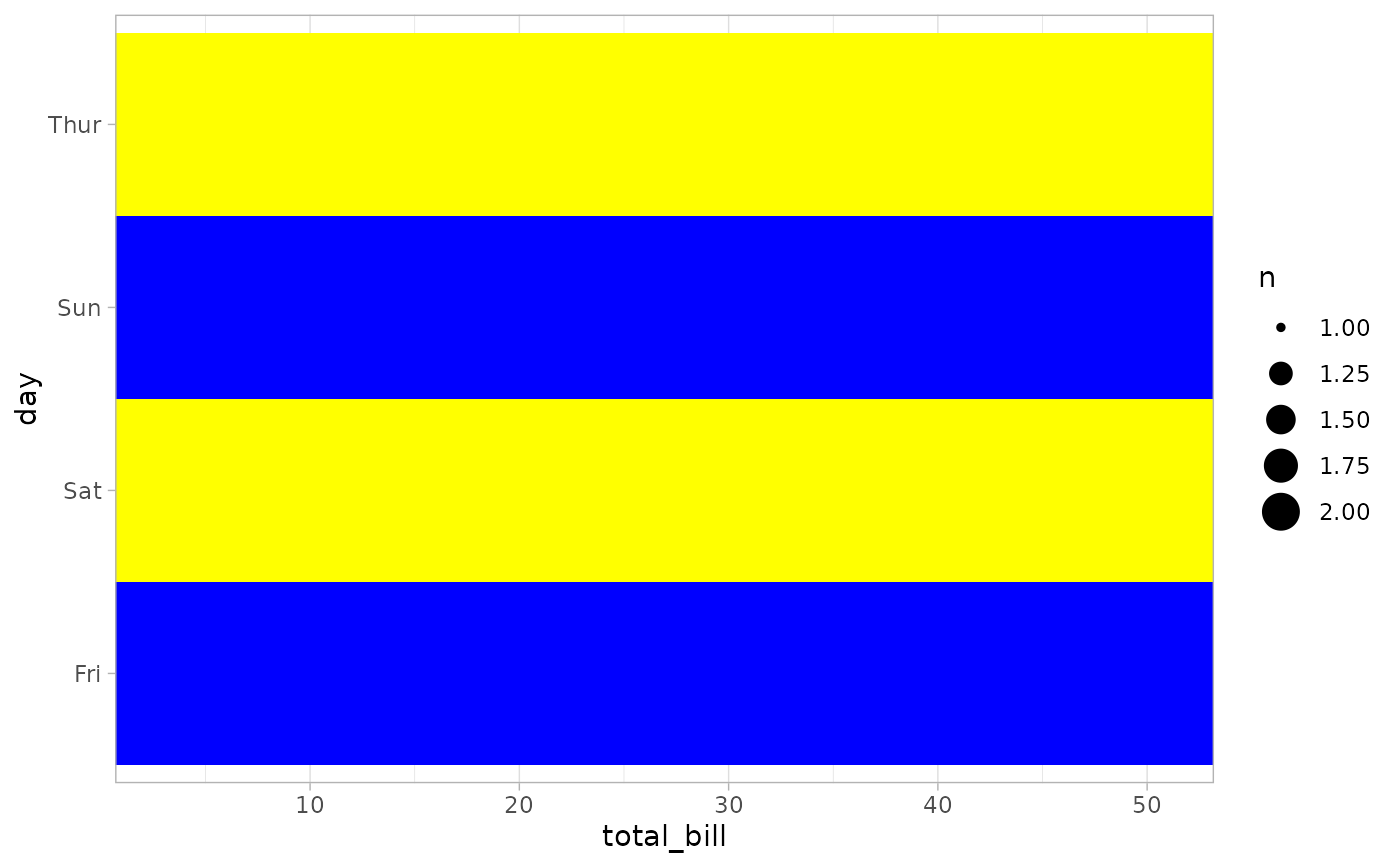p + geom_stripped_rows(odd = "blue", even = "yellow", alpha = .1)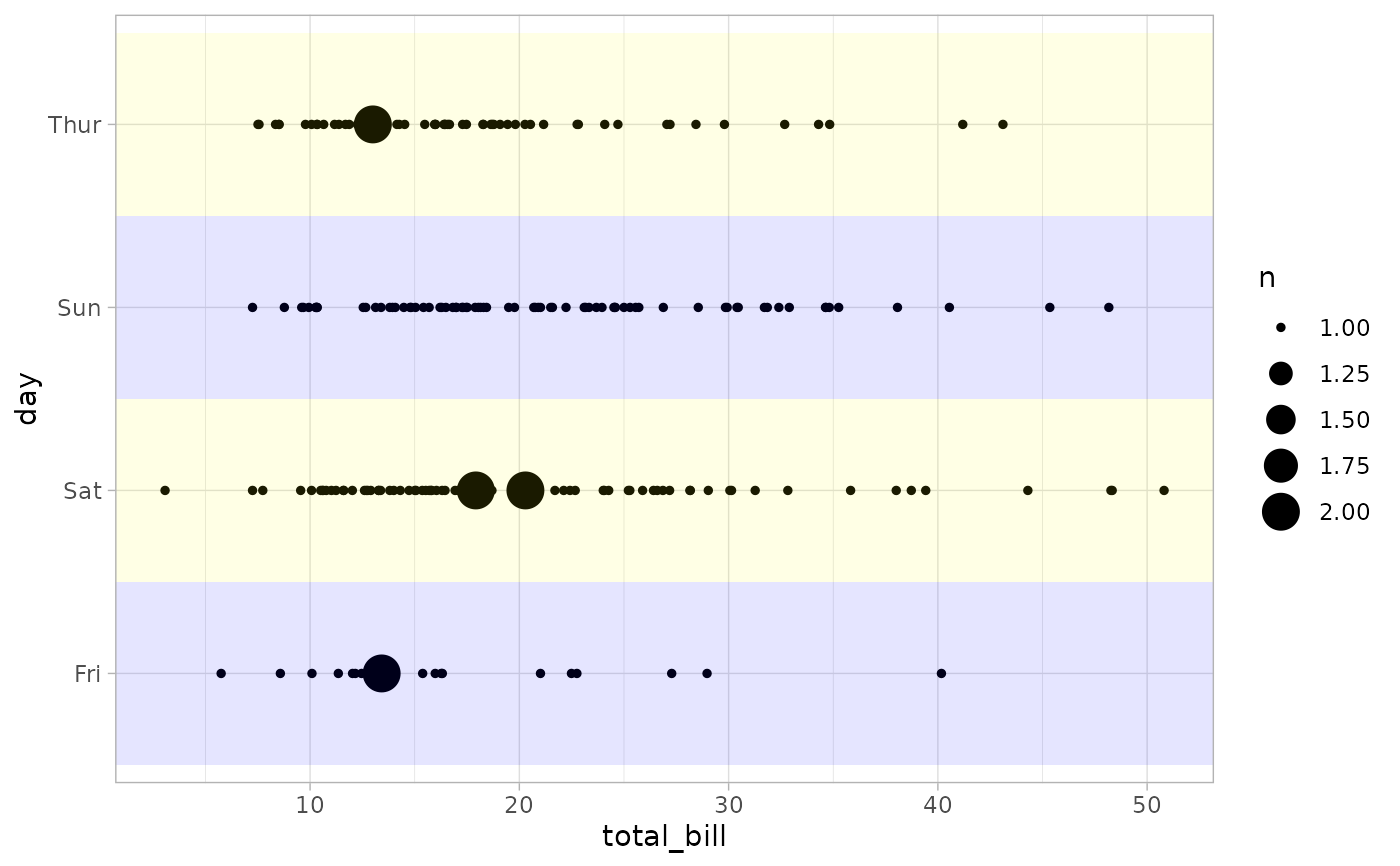p + geom_stripped_rows(odd = "#00FF0022", even = "#FF000022")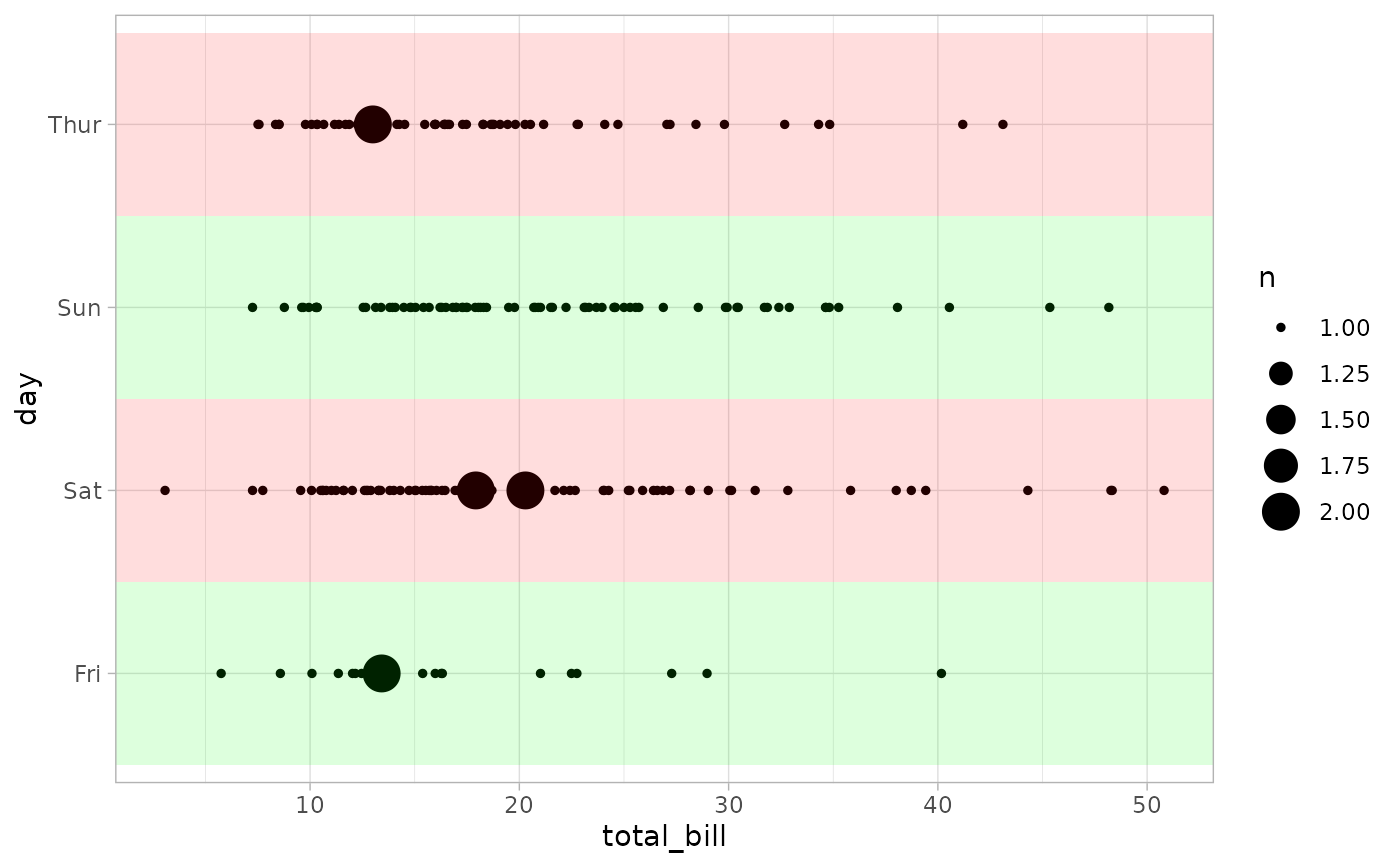p + geom_stripped_cols()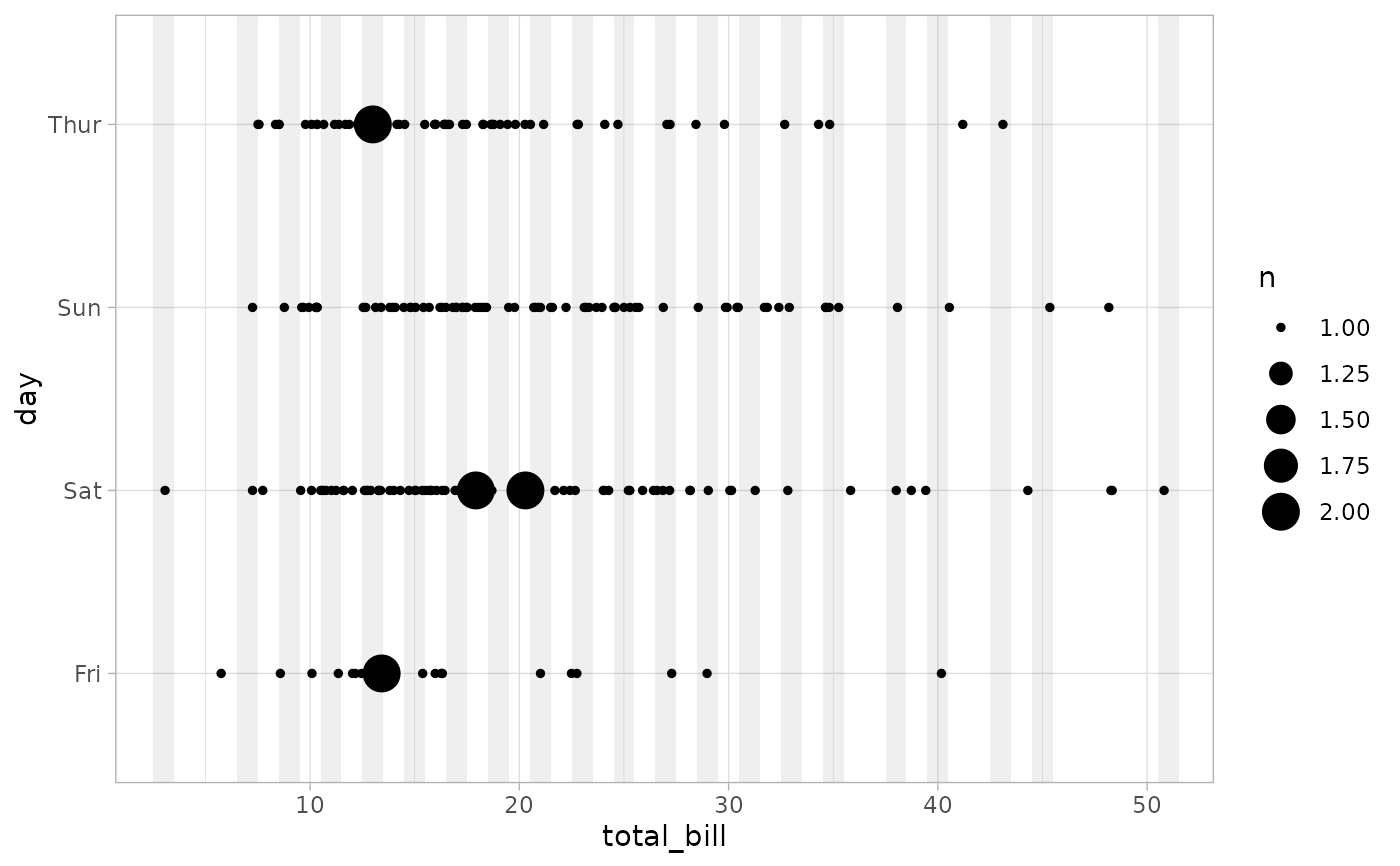p + geom_stripped_cols(width = 10)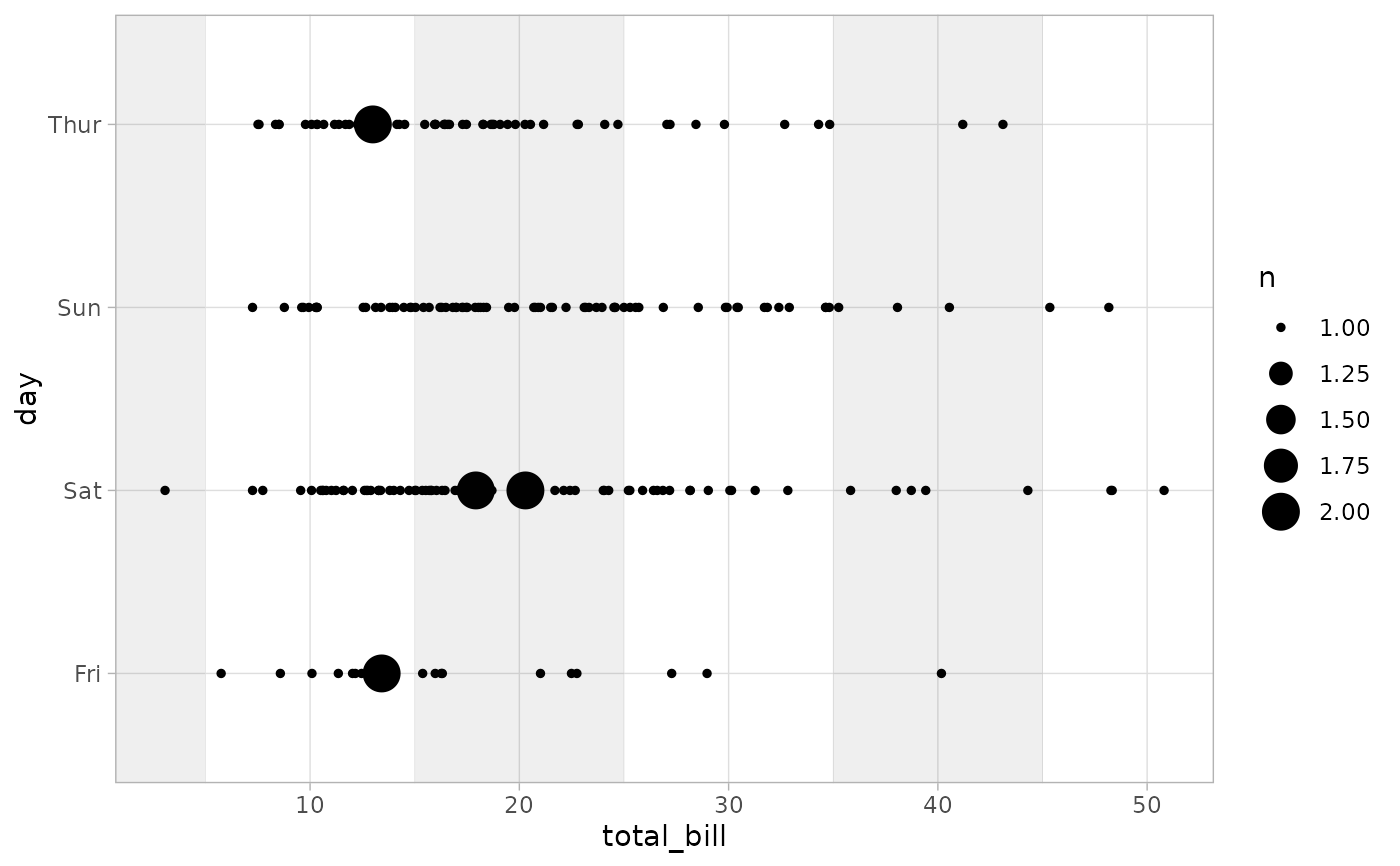p + geom_stripped_cols(width = 10, nudge_x = 5)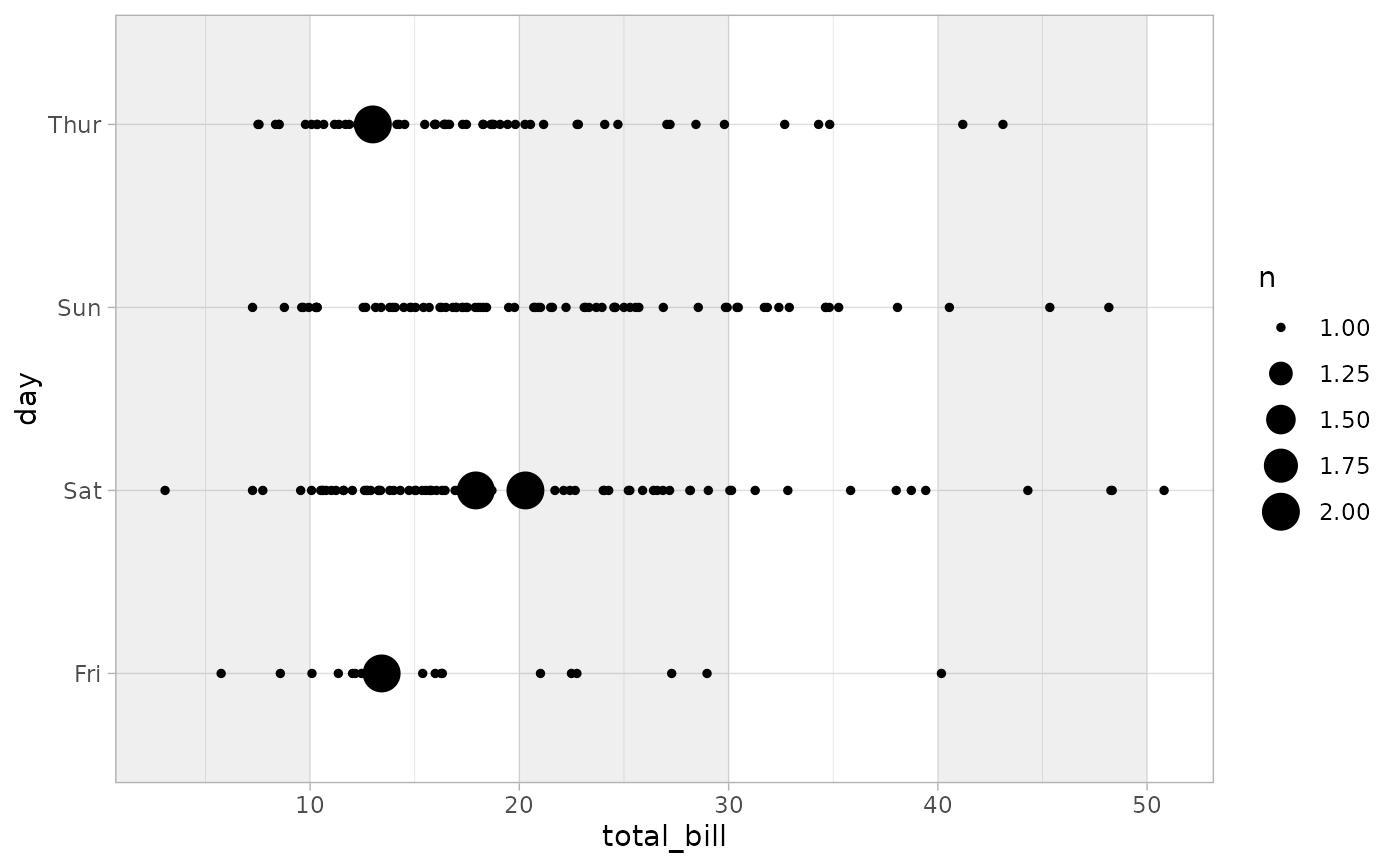# }